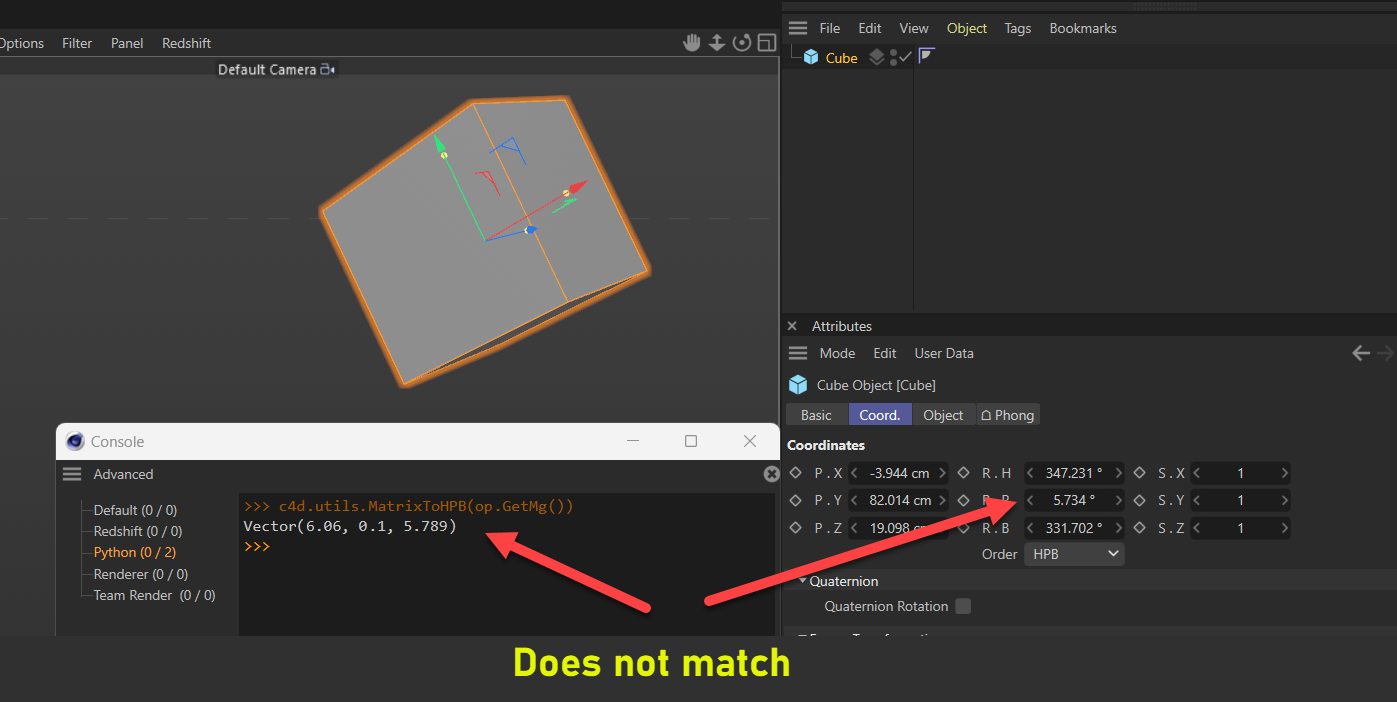# Solved MatrixToHPB Not Giving the Global Rotation?

Hi,

Based on the forums, the way to get the Global Rotation is
`c4d.Utils.MatrixtoHPB(op.GetMg())`

But it somehow gives different values. In the image below, the local rotation should also be the global since there is no parent.

But as you can see, it does maatch the figure in the console.

Is there a way around this?Aw.
My bad. It is on radians. I have to ran this command.
`c4d.utils.RadToDeg()`

Nothing to see here folks lol

Hello @bentraje,

thank you for reaching out to us. There is nothing much left to add for us here. All angle values are represented internally in radians in Cinema 4D, only the UI does convert to degrees. Except for displaying angle values in UIs, I would however recommend to embrace the unit of radians in code rather than converting in and out of it.

There is no computional downside to always doing the conversions, e.g., `theta: float = c4d.utils.DegToRad(90)`. But writing something like `theta: float = math.pi * .5` forces oneself to understand the intimate relation between a trigangle and the unit circle, i.e., the circle or trigonometric functions. Understanding radians and why it is a useful unit is then an emergent property of that understanding of trigonometry.

Cheers,
Ferdinand

MAXON SDK Specialist
developers.maxon.net